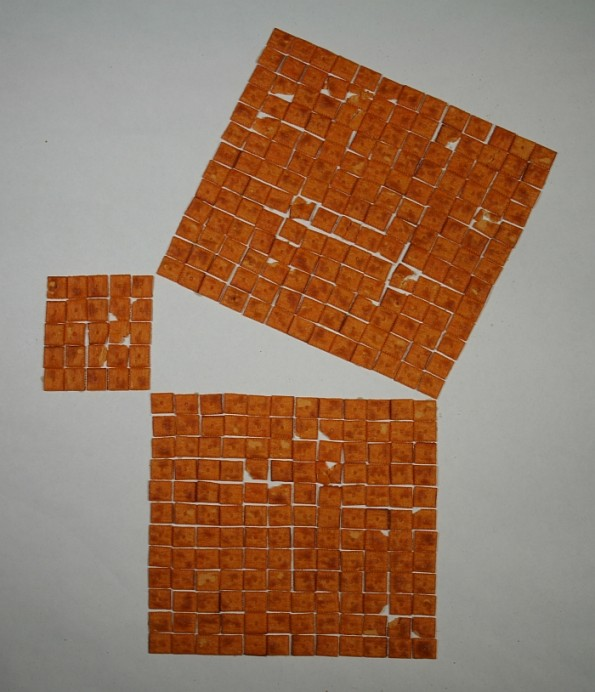Math Monday: Math-Play With Your Food
MARCH 15, 2010

by George Hart

Making things with your food is an age-old pastime. Here are two mathematical constructions made from crackers.This illustrates the Pythagorean Theorem for a 5-12-13 right triangle. The number of crackers in the two small squares (25+144) equals the number of crackers in the square on the hypotenuse (169).With triangular crackers, this 3-4-5 right triangle illustrates a more general idea. The number of crackers in the two small triangles (9+16) equals the number of crackers in the large triangle (25). Here is an introduction to the Pythagorean Theorem with two simple proofs, and here is a detailed explanation of why this generalization works.